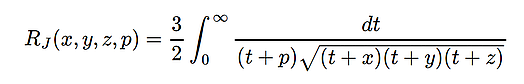## complete

• the complete elliptic integral of the first kind

• elliptic_k(m)
• elliptic_k2(m) == elliptic_k(m^2)• the complete elliptic integral of the second kind

• elliptic_e(m)
• elliptic_e2(m) == elliptic_e(m^2)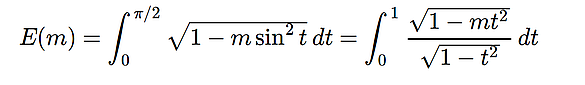• the complete elliptic integral of the third kind

• elliptic_pi(n, m)
• elliptic_pi2(n, m) == elliptic_pi(n, m^2)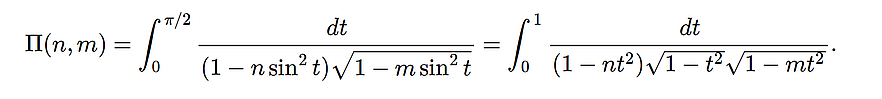## incomplete

• the incomplete elliptic integral of the first kind

• elliptic_f(phi, m)
• elliptic_f2(phi, m) == elliptic_f(phi, m^2)• the incomplete elliptic integral of the second kind

• elliptic_e(phi, m)
• elliptic_e2(phi, m) == elliptic_e(phi, m^2)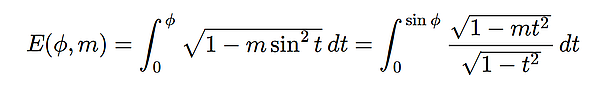• the incomplete elliptic integral of the third kind

• elliptic_pi(n, phi, m)
• elliptic_pi2(n, phi, m) == elliptic_pi(n, phi, m^2)## symmetric (Carlson)

• the symmetric elliptic integral of the first kind
• elliptic_rf(x, y, z)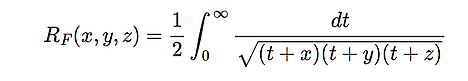• the symmetric elliptic integral of the second kind
• elliptic_rg(x, y, z)• the symmetric elliptic integral of the third kind
• elliptic_rj(x, y, z)